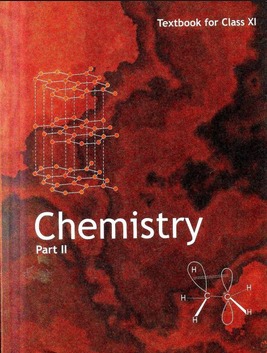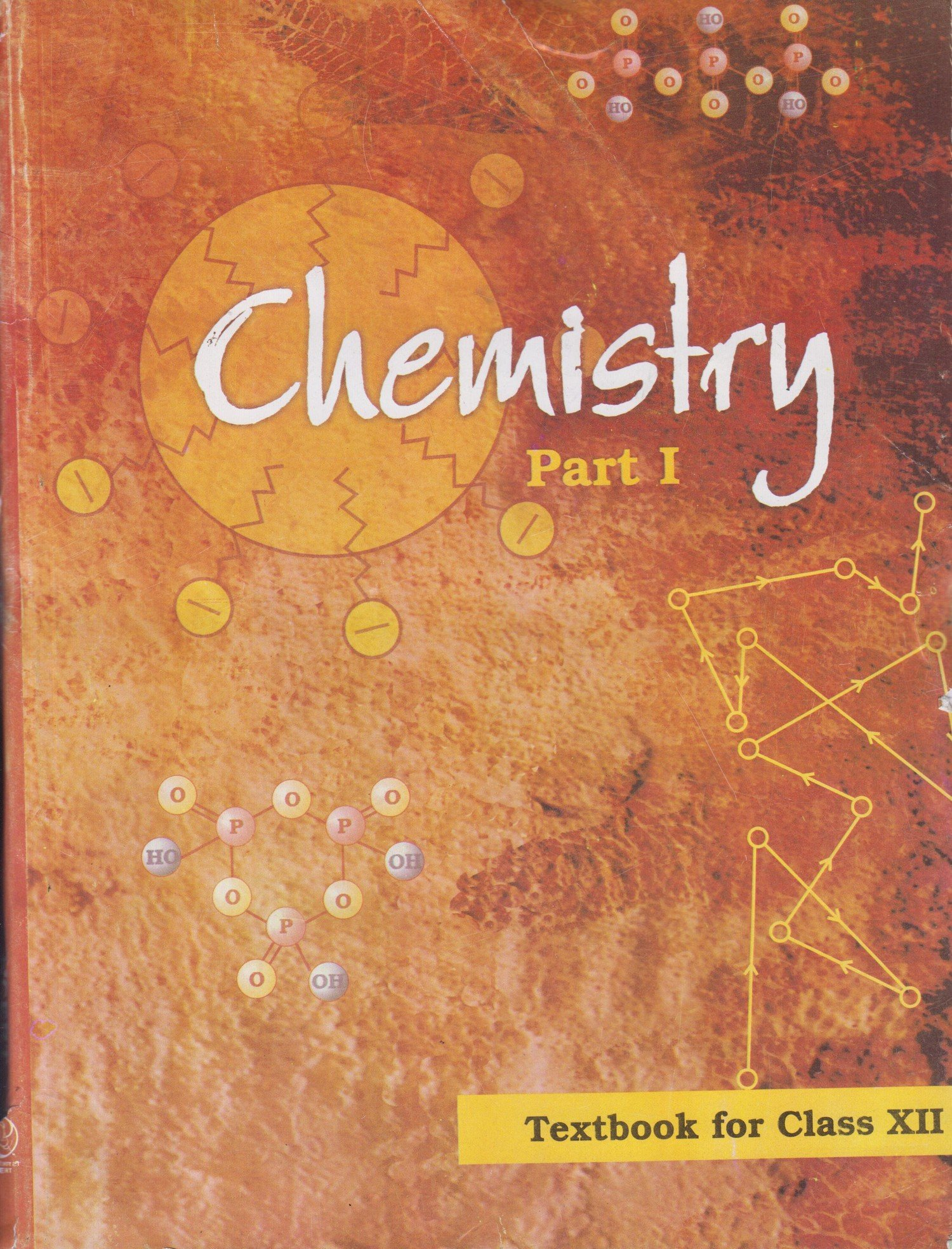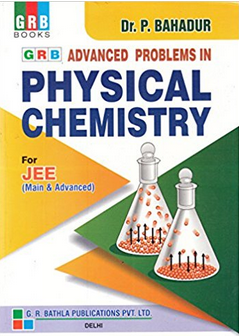# Redox Reaction and Electrochemistry   Share

## What is Redox Reaction and Electrochemistry

Electrochemistry: It is the study of the production of electricity from the energy released during spontaneous chemical reactions and the use of energy as non-spontaneous chemical transformations.

Redox reaction: These are oxidation and reduction reaction that describes all chemical reactions in which the oxidation number is decreased or increased. The redox contains two kinds of concepts i.e, reduction and oxidation. They can be explained in simple terms :

• Oxidation is the loss of electrons by a molecule, atom, or ion

• The reduction in the gain of electrons by a molecule, atom, or ion.

ElectrolysisElectrolysis experiment drives a nonspontaneous chemical reaction to occur. This is obtained when two electrodes are immersed in an electrically conductive solution.

Aqueous solutions of salts can be electrolyzed as well because they are also electrically conductive.in aqueous, there is an additional reaction possible at each anode and cathode.

CATHODE: water will be reduced if the cation is very active metal. Very active metals like Li, Sr, Ba, Ca, Cs, Na, K. If the cation is active or inactive metal the cation will be reduced.

ANODE: Water will mostly oxidize if the anion is polyatomic, water is oxidized during the process when there is a presence of sulfate, perchlorates, nitrate ions are deoxidized. water is deoxidized during the process when there is the presence of chloride, bromide and iodide ions are oxidized.

There are some various real-life applications that we see in our daily life around us. Some of them are mentioned below:

• Nowadays, lithium-ion batteries are gaining a lot of attention and it is widely used in laptops, mobiles, automobiles, drones, etc.• The reactions carried out electrochemically can be energy efficient and less polluting.• AED: Automated External Defibrillator. This is the device which is used to give a shock through the chest to the heart. This shock resumes the normal rhythm.## Notes for Redox Reaction and Electrochemistry

In this section, you will study about the important topics of the chapter, overview, formulae and some important tips and guidelines for the preparation of the chapter at the best.

## Important Topics of Redox Reaction and Electrochemistry

• Electrochemical cells
• Galvanic cells
• Nernst equation
• The conductance of electrolytic solutions
• Electrolytic cells and electrolysis
• Batteries
• Fuel cells
• Corrosion

## Overview of the Chapter- Redox Reaction and Electrochemistry

GALVANIC CELLS:  when we place copper metal into an AgNO3 solution, a redox reaction occurs.
2Ag+(aq) +Cu(s) 2Ag(s) + Cu2+(aq)

• Above reaction stops when copper metal gets covered with silver.

• For extraction of energy from spontaneous reactions we need to separate the two half-reactions by building a GALVANIC CELL.

• Anode: negative (electrons left by Cu oxidation)

• Cathode: positive (Ag+becomes part of the electrode)

• The standard state for the galvanic cell is a pressure of 1 atm for all gases, at a temperature of 298 kelvin(K) and concentration of 1 Molarity (M) for all soluble compounds, liquids, and solids.Nernst Equation: Nernst equation was given to find the cell potential of any reaction. For a general electrochemical reaction as follows:

Nernst equation can be written as follows:

The Equilibrium constant from Nernst Equation:  After a certain amount of time, this electrochemical reaction reaches equilibrium and this equilibrium constant can be calculated from the Nernst equation as follows:

This equation gives the relationship between the equilibrium constant and the cell potential. Thus, it is an easy way to calculate the equilibrium constant for the electrochemical reaction from the cell potential.

## You must from your physics syllabus that the resistance of any material is given as follows: where l is the length of the material, A is its cross-section and ρ is the resistivity. In the same way, conductance is the inverse of resistance and it is given as follows: The SI unit of conductance is siemens and it is represented by 'S'.

Measurement of conductivity of Ionic solution:

Balancing redox reactions:

• This results in a change in oxidation number.

• OXIDATION (reducing agent): loss of electrons

• REDUCTION (oxidizing agent): gain of electronsEx: combustion of methane
C + H4(g) + 2O2(g) <=> C O2(g) + 2H2 O(l)

• Balance as if under acidic conditions.

• For each H+in the final equation adds, OH- to both sides.

• Combine H+ and OH-  to produce H2O.

• Cancel H2O from both sides if needed.

Standard reduction potentials:

• Due to the difference in electrical potential energy, the flow of electrical current from anode to cathode between the electrodes takes place.

• Measured using a device called “VOLTMETER”.

• Units volts (V).
The measured current is called overall CELL POTENTIAL(Ecell)_

• Is a function of concentration ,temperature , metals/ions used.

• If Voltage (+ ve) ,the reaction occurs spontaneously.

• if Voltage (-ve), the reaction does not occur spontaneously.

Standard cell potential:

• The cell potential at standard state conditions,
[ions] =1 M, T=25C, 1 atm gas pressure.

• Each half-reaction is prescribed to have a reduction potential associated with it, that itself provides the tendency of half-reaction to proceed as a reduction.

Standard reduction potential [E]

• When the two half-reactions are connected :

• The reduction process is under governed by one with larger standard reduction potential (more positive ) Ered (cathode).

• The oxidation process is under governed by one with lesser Ered(anode).
Eox = -(Ered)
The final equation is as follows:
Ecell= Ered + Eox

• By this, we can only measure Ecell, in order to find for Ered and Eox we have to select a specific half cell as our reference.

Reference half cell:

• Standard hydrogen electrode(SHE).

• At standard state conditions, it assigned a value of 0 V.

• The reaction is as follows:
2H+(1M) +2e- H2(1 atm)

Batteries:

• The battery is a galvanic cell, that contains the source of electric current. It also can be a series of galvanic cells.

• Made in the series connection of 6 identical batteries( each of 2V)

• This is rechargeable using electrolysis and during discharge it uses H2SO4.

• It contains lead as anode and PbO2 as a cathode, they are immersed in H2SO4.

• It contains lead as anode and PbO2 as a cathode, they are immersed in H2SO4.

CORROSION:

• It is a naturally occurring process in which it converts a refined material to a more chemically stable form, such as hydroxide, oxide, sulfide.

• It results in the gradual destruction of metals by chemical or electrochemical reaction with their environment.

• Ex: rusting of iron or steel, copper oxide.

• Prevented by the formation of alloy, coating with a layer of less active metal, passivation, by cathodic protection.

## How to prepare for Redox Reaction and Electrochemistry?

• This chapter is a part of Physical chemistry. This chapter is one of the most important chapters of the complete chemistry syllabus. Its concepts, laws, numerical and graphs all are important both for the basic foundation of chemistry and for scoring good marks in the examination.

• Before reading this chapter, first, you must have the basic knowledge of the mole concept.

• You must learn carefully how to balance and various methods for balancing the chemical equations, Nernst equation, the conductance of electrolytic solutions.

• Rest this chapter is very simple, just be regular and be consistent in your numerical practice.

## Prescribed Books for Redox Reaction and electrochemistry

First, you must finish the class XI NCERT textbook and solve each and every example and unsolved question given in it. Then for advanced level preparation like JEE and NEET, you must follow R.C. Mukherjee and O.P. Tandon. You must definitely solve the previous year papers. Meanwhile, in the preparation, you must continuously write the mock tests for the depth of knowledge. Our platform will help you to provide with the variety of questions for deeper knowledge with the help of videos, articles and mock tests.

## Chemistry Chapter- wise Notes for Engineering and Medical Exams

 Chapters No. Chapters Name Chapter 1 Some basic concepts in chemistry Chapter 2 States of matter Chapter 3 Atomic Structure Chapter 4 Solutions Chapter 5 Chemical Thermodynamics Chapter 6 Equilibrium Chapter 8 Chemical kinetics Chapter 9 Surface Chemistry Chapter 10 General Principle and processes of Isolation of metals Chapter 11 Classification of Elements and Periodic table Chapter 12 Hydrogen Chapter 13 p- block Elements Chapter 14 s-block Elements (Alkali and Alkaline Earth Metals) Chapter 15 Chemical Bonding and Molecular Structure Chapter 16 d- and f- BLOCK ELEMENTS Chapter 17 Coordination Compounds Chapter  18 Environmental Chemistry Chapter 19 Purification and Characterisation of Organic Compounds Chapter 20 Some Basic Principles of Organic Chemistry Chapter 21 Hydrocarbons Chapter 22 Organic Compounds containing Halogens Chapter 23 Organic Compounds containing Oxygen Chapter 24 Organic Compounds Containing Nitrogen Chapter 25 Polymers Chapter 26 Biomolecules Chapter 27 Chemistry in Everyday Life Chapter 28 Principles Related to Practical Chemistry

### Topics from Redox Reaction and Electrochemistry

• Redox reactions ( JEE Main, MET, GUJCET Pharmacy ) (15 concepts)
• Electronic concepts of oxidation and reduction ( JEE Main, MET, GUJCET Pharmacy ) (12 concepts)
• Half - cell and cell reactions ( JEE Main, MET, GUJCET Pharmacy ) (8 concepts)
• Oxidation number, rules for assigning oxidation number ( JEE Main, MET, GUJCET Pharmacy ) (16 concepts)
• Balancing of redox reactions ( JEE Main, MET, GUJCET Pharmacy ) (3 concepts)
• Different types of electrodes, electrode potentials including standard electrode potential ( JEE Main, MET, GUJCET Pharmacy ) (6 concepts)
• Conductance in electrolytic solutions ( JEE Main, MET, GUJCET Pharmacy ) (22 concepts)
• Nernst equation and its applications ( JEE Main, MET, GUJCET Pharmacy ) (4 concepts)
• Relationship between cell potential and Gibbs' energy change ( JEE Main, MET, GUJCET Pharmacy ) (16 concepts)
• Eectrolytic and metallic conduction ( JEE Main, MET, GUJCET Pharmacy ) (2 concepts)
• Electrochemical cells - Electrolytic and Galvanic cells ( JEE Main, MET, GUJCET Pharmacy ) (2 concepts)
• Molar conductivities and their variation with concentration ( JEE Main, MET, GUJCET Pharmacy ) (12 concepts)
• Kohlrausch's law and its applications ( JEE Main, MET, GUJCET Pharmacy ) (6 concepts)
• OXIDATION NUMBER AND OXIDATION STATE ( JEE Main, MET, GUJCET Pharmacy ) (1 concepts)
• TYPES OF REDOX REACTIONS ( JEE Main, MET, GUJCET Pharmacy ) (2 concepts)
• BALANCING OF REDOX REACTIONS ( JEE Main, MET, GUJCET Pharmacy ) (3 concepts)
• ELECTROCHEMICAL CELLS ( JEE Main, MET, GUJCET Pharmacy ) (4 concepts)
• GALVANIC CELLS ( JEE Main, MET, GUJCET Pharmacy ) (7 concepts)
• NERNST EQUATION ( JEE Main, MET, GUJCET Pharmacy ) (5 concepts)
• CONDUCTANCE OF ELECTROLYTIC SOLUTIONS ( JEE Main, MET, GUJCET Pharmacy ) (4 concepts)
• KOHLRAUSCH'S LAW ( JEE Main, MET, GUJCET Pharmacy ) (1 concepts)
• BATTERIES ( JEE Main, MET, GUJCET Pharmacy ) (2 concepts)

### Important Books for Redox Reaction and Electrochemistry

•••Exams
Articles
Questions